# Abstract Algebra : Groups

## Example Questions

### Example Question #1 : Abstract Algebra

Which of the following is an identity element of the binary operation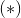?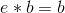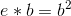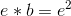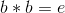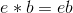Explanation:

Defining the binary operationwill help in understanding the identity element. Sayis a set and the binary operator is defined as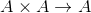for all given pairs in.

Then there exists an identity element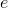insuch that given,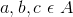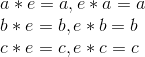### Example Question #1 : Introduction

Which of the following illustrates the inverse element?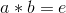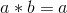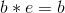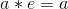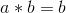Explanation:

For every element in a set, there exists another element that when they are multiplied together results in the identity element.

In mathematical terms this is stated as follows.

For every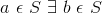such thatwhere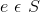andis an identity element.

### Example Question #1 : Abstract Algebra

identify the following definition.

Givenis a normal subgroup of, it is denoted that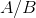when the group of left cosets ofinis called __________.

Subgroup

Cosets

Simple Group

Normal Group

Factor Group

Factor Group

Explanation:

By definition of a factor group it is stated,

Givenis a normal subgroup of, it is denoted thatwhen the group of left cosets ofinis called the factor group ofwhich is determined by.

### Example Question #4 : Abstract Algebra

Determine whether the statement is true of false: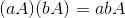True

False

True

Explanation:

This statement is true based on the following theorem.

For allin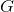.

Ifis a normal subgroup ofthen the cosets offorms a group under the multiplication given by,### All Abstract Algebra Resources